SSC  >  SSC CPO Mock Test (Quant Apt) 5

# SSC CPO Mock Test (Quant Apt) 5

Test Description

## 50 Questions MCQ Test SSC CPO & Constable - Mock Tests & Previous Year Papers | SSC CPO Mock Test (Quant Apt) 5

SSC CPO Mock Test (Quant Apt) 5 for SSC 2023 is part of SSC CPO & Constable - Mock Tests & Previous Year Papers preparation. The SSC CPO Mock Test (Quant Apt) 5 questions and answers have been prepared according to the SSC exam syllabus.The SSC CPO Mock Test (Quant Apt) 5 MCQs are made for SSC 2023 Exam. Find important definitions, questions, notes, meanings, examples, exercises, MCQs and online tests for SSC CPO Mock Test (Quant Apt) 5 below.
Solutions of SSC CPO Mock Test (Quant Apt) 5 questions in English are available as part of our SSC CPO & Constable - Mock Tests & Previous Year Papers for SSC & SSC CPO Mock Test (Quant Apt) 5 solutions in Hindi for SSC CPO & Constable - Mock Tests & Previous Year Papers course. Download more important topics, notes, lectures and mock test series for SSC Exam by signing up for free. Attempt SSC CPO Mock Test (Quant Apt) 5 | 50 questions in 30 minutes | Mock test for SSC preparation | Free important questions MCQ to study SSC CPO & Constable - Mock Tests & Previous Year Papers for SSC Exam | Download free PDF with solutions
 1 Crore+ students have signed up on EduRev. Have you?
SSC CPO Mock Test (Quant Apt) 5 - Question 1

### The average age of a husband and wife was 23 years at the time of their marriage. After 5 years they have a one year old child. The average age of the family now is

Detailed Solution for SSC CPO Mock Test (Quant Apt) 5 - Question 1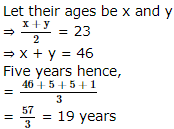SSC CPO Mock Test (Quant Apt) 5 - Question 2

### Nine persons went to a hotel for taking their meals. Eight of them spent Rs 12 each over their meals and the ninth spent Rs 8 more than the average expenditure of all the nine. Total money spent by them was

Detailed Solution for SSC CPO Mock Test (Quant Apt) 5 - Question 2

Let the average expenditure of all the nine be Rs. x.
Then. 12 × 8 + (x + 8) = 9x
⇒ x = 13
Total money spent = 9x = 9 × 13 = Rs. 117

SSC CPO Mock Test (Quant Apt) 5 - Question 3

### If 1/x = 3 and y = 4, then what is x in terms of y?

Detailed Solution for SSC CPO Mock Test (Quant Apt) 5 - Question 3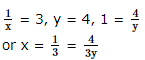SSC CPO Mock Test (Quant Apt) 5 - Question 4

If α and β are the two roots of the equation 2x2-7x-3=0, then find the value of (α+2)(β+2)

SSC CPO Mock Test (Quant Apt) 5 - Question 5
The price of 3 table-fans and 3 ceiling-fans is Rs 4,350.If the cost of 2 ceiling-fans and 1 table-fan is Rs 2,250,what is the cost of one ceiling fan?
Detailed Solution for SSC CPO Mock Test (Quant Apt) 5 - Question 5 Let price of one table fan = Rs x
and price of one ceiling fan = Rs y
So 3x + 3y = 4350 .....(1)
x + 2y = 2250 .....(2)
Multiplying 2 in equation (1) and 6 in equation (2) we get
6x + 6y = 8700 .....(3)
6x + 12y = 13500 .....(4)
Subtracting equation (3) from equation (4) we get
6y = 4800
⇒ y = 800
So cost of one ceiling fan Rs 800
SSC CPO Mock Test (Quant Apt) 5 - Question 6

The minute hand and the hour hand of a clock are 14 cm and 7 cm long respectively. The total distance covered by their tips in one day is

Detailed Solution for SSC CPO Mock Test (Quant Apt) 5 - Question 6

Distance Travel by hour hand in one day is = 2 × 2 πr
= 2 × 2 × (22/7) × 7 = 88 cm
Distance Travel by minute hand in one day is = 2 πr × 24
= 2 × (22/7) × 14 × 24 = 2112 cm
Total distance covered by their tips is = 2112 + 88 = 2200 cm = 22m

SSC CPO Mock Test (Quant Apt) 5 - Question 7
The equation of a st. line passing thro' the point (-5, 4) and which cuts off an intercept of √2 units between the lines
x + y + 1 = 0 and x + y - 1 = 0 is
SSC CPO Mock Test (Quant Apt) 5 - Question 8

The wheel of a scooter has a diameter of 70 cm. The number revolution it must make to maintain a speed of 33 km/hour is

Detailed Solution for SSC CPO Mock Test (Quant Apt) 5 - Question 8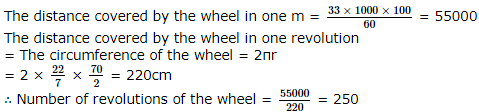SSC CPO Mock Test (Quant Apt) 5 - Question 9

A sum invested for 2 years 4 months at the rate of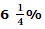pa compounded annually will amount to Rs. 14,641. The sum is closest to

Detailed Solution for SSC CPO Mock Test (Quant Apt) 5 - Question 9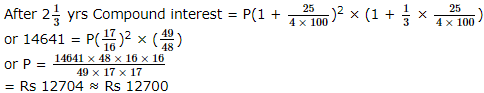SSC CPO Mock Test (Quant Apt) 5 - Question 10
The average of 20 numbers is 0. Of them atmost how many can be greater than zero?
SSC CPO Mock Test (Quant Apt) 5 - Question 11

A park is in form of a rectangle 120m x 100m. At the centre of the park is a circular lawn. The area of the park excluding the lawn is 10614 m2. The cost of grass carpeting the lawn @ Rs. 27/m2 is

Detailed Solution for SSC CPO Mock Test (Quant Apt) 5 - Question 11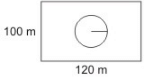Area of the park = 100 × 120
= 12000 m2
Area of the park excluding the lawn = 10614 m2
∴ The Area of the lawn = 12000 - 10614 = 1386 m2
The Cost of fencing the lawn = Rs. (27 × 1386) = Rs. 37422.00

SSC CPO Mock Test (Quant Apt) 5 - Question 12

If the arithmetic mean (A.M) of seventy five numbers is calculated, it is 35. If each number is increased by 5, then A.M.of new numbers is

Detailed Solution for SSC CPO Mock Test (Quant Apt) 5 - Question 12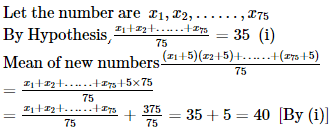SSC CPO Mock Test (Quant Apt) 5 - Question 13
A shopkeeper marks his goods 50% more than their cost price and them allows a discount of 20%. His gain or loss percent is
SSC CPO Mock Test (Quant Apt) 5 - Question 14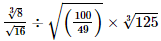is equal to

SSC CPO Mock Test (Quant Apt) 5 - Question 15

Evaluate :[((2.39)²-(1.61)²)/((2.39)-(1.61))]

SSC CPO Mock Test (Quant Apt) 5 - Question 16

In the given figure AD and AF are tangents to the circle. Then the correct statement out of the following is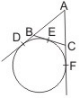Detailed Solution for SSC CPO Mock Test (Quant Apt) 5 - Question 16

BE = BD and CE = CF
pr. ∆ABC = AB + BE + CE + CA
= AB + BC + CF + CA

SSC CPO Mock Test (Quant Apt) 5 - Question 17

Determine the value of ∠AOE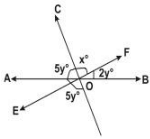SSC CPO Mock Test (Quant Apt) 5 - Question 18
If in a parallelogram, the diagonals are equal and perpendicular, then it is a
SSC CPO Mock Test (Quant Apt) 5 - Question 19

All sides of a polygon of 36 sides are equal. Each of its interior angle is of mesure

Detailed Solution for SSC CPO Mock Test (Quant Apt) 5 - Question 19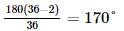SSC CPO Mock Test (Quant Apt) 5 - Question 20
The HCF of two numbers is 8. Which one of the following can never be their LCM?
SSC CPO Mock Test (Quant Apt) 5 - Question 21

The angle of elevation of an object from a point P on the level ground is α . Moving d metres on the ground towards the objcet, the angle of elevation is found to be β . Then the height (in metres) of the object is

SSC CPO Mock Test (Quant Apt) 5 - Question 22

A number when divided by 32 leaves the remainder 29.This number when divided by 8 will leave the remainder

Detailed Solution for SSC CPO Mock Test (Quant Apt) 5 - Question 22

Let x be the number and y be the quotient
Then x = 32 × y + 29
= (8 × 4 × y) + (8 × 3) + 5
= 8 × (4y + 3) + 5
Hence required remainder is 5

SSC CPO Mock Test (Quant Apt) 5 - Question 23
A candidate who gets 20% marks in examination fails by 30 marks but another candidate who gets 32% gets 42 marks more than the pass marks.Then the percentage of pass marks is
SSC CPO Mock Test (Quant Apt) 5 - Question 24

In a certain shop, which stocks four types of caps,there are 1/3 as many red caps as blue and 1/2 as many green caps as red caps. There are 42 blue caps, then what percent of the total caps in the shop are blue?

Detailed Solution for SSC CPO Mock Test (Quant Apt) 5 - Question 24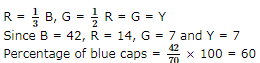SSC CPO Mock Test (Quant Apt) 5 - Question 25

If 5% more is gained by selling an article for Rs 350, then by selling it for Rs 340,the cost of the article is

Detailed Solution for SSC CPO Mock Test (Quant Apt) 5 - Question 25

Let C.P. be Rs x
Then, 5% of x = (350 - 340) = 10
⇒ x/20 = 10
⇒ x = 200

SSC CPO Mock Test (Quant Apt) 5 - Question 26
A tap can empty a tank in one hour. A second tap can empty it in 30 minutes. If both the taps operate simultaneouslyy, how much time is needed to empty the tank?
SSC CPO Mock Test (Quant Apt) 5 - Question 27
The ratio between the sale price and the cost price of an article is 7:5. What is the ratio between the profit and the cost price of that article?
SSC CPO Mock Test (Quant Apt) 5 - Question 28
If data given to construct a triangle ABC are a = 5, b = 7, sin A = 3/4, then it is possible to construct :
SSC CPO Mock Test (Quant Apt) 5 - Question 29
The ratio of the numbers of boys and girls of a school with 504 students is 13:11. What will be the new ratio if 12 more girls are admitted?
SSC CPO Mock Test (Quant Apt) 5 - Question 30
Two liquids A and B are in the ratio 5 : 1 in container I and 1 : 3 in container II. In what ratio should the contents of the two containers be mixed so as to obtain a mixture of A and B in the ratio 1 : 1?
SSC CPO Mock Test (Quant Apt) 5 - Question 31
The two geometric means between 1 and 64 are
Detailed Solution for SSC CPO Mock Test (Quant Apt) 5 - Question 31 The geometric progression is 1, c2, c3, 64
a = 1, c4 = ar4 - 1 = 1(r)3 = 64
Thus r = 4
c2 = 4 and c3 = 4.4 = 16
The two geometric means are 4 and 16
SSC CPO Mock Test (Quant Apt) 5 - Question 32
The simple interest on a sum of money is 1/4 of the principal. If number of yrs is equal to rate % per annum, the interest rate is
SSC CPO Mock Test (Quant Apt) 5 - Question 33
The least number that must be added to 2887 so that the sum may be a perfect square is
SSC CPO Mock Test (Quant Apt) 5 - Question 34

A train 110 m long takes 3 seconds to pass a standing man. How long is the platform if the train passes through it in 15 seconds moving with the same speed?

Detailed Solution for SSC CPO Mock Test (Quant Apt) 5 - Question 34

Speed of the train = 110/3 m/s
∴ Length of the platform
= Speed of train x Time taken to pass the platform
= ( 110/3 x 15) m = 550 m

SSC CPO Mock Test (Quant Apt) 5 - Question 35
If a man can cut the grass of a lawn in M minutes, what part of the lawn\'s grass can be cut in 25 minutes?
SSC CPO Mock Test (Quant Apt) 5 - Question 36
If sin (π cosθ)=cos(π sinθ), then the value of cos (θ+π/4)
SSC CPO Mock Test (Quant Apt) 5 - Question 37

cos 40°+cos 80°+cos 160°+cos 240°

SSC CPO Mock Test (Quant Apt) 5 - Question 38
If A+B+C=π, then cos2A+cos2B+cos2C=
SSC CPO Mock Test (Quant Apt) 5 - Question 39

Water flows through a cylindrical pipe of internal diameter 7 cm at 2 m per second. If the pipe is always half full, then what is the volume of water (in litres) discharged in 10 minutes?

Detailed Solution for SSC CPO Mock Test (Quant Apt) 5 - Question 39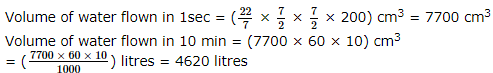SSC CPO Mock Test (Quant Apt) 5 - Question 40
If a metallic cuboid weighs 16 kg, how much would a miniature cuboid of metal weigh, if all dimensions are reduced to one-fourth of the original?
SSC CPO Mock Test (Quant Apt) 5 - Question 41

A cube of edges 5 cm is cut into cubes each of edge 1 cm. The ratio of the total surface area of one of the small cubes to that of the large cube is equal to :

Detailed Solution for SSC CPO Mock Test (Quant Apt) 5 - Question 41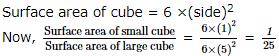SSC CPO Mock Test (Quant Apt) 5 - Question 42

In a school the periodical examinations are held every second month. In a session during April 2001-March 2002, a student of Class IX appeared for each of the perodical exams. The aggregate marks obtained by him in each periodical exam are represented in the line graph given below. Study the graph and answer the question based on it given below.
Marks obtained by a student in six periodical exams held in every two months during the years in the session 2001-02. Maximum total marks in each periodical exam = 500.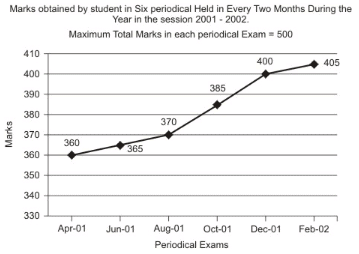Q. The total number of marks obtained in Feb. 02 is what per cent of the total marks obtained in Apr. 01 ?

Detailed Solution for SSC CPO Mock Test (Quant Apt) 5 - Question 42

From the graph that the student obtained 360, 365, 370, 385, 400 and 405 marks in periodical exams held in Apr 01, Jun 01, Aug 01, Oct 01, Dec 01 and Feb 02 respectively.
Required percentage = (405/360 × 100) % = 112.5%

SSC CPO Mock Test (Quant Apt) 5 - Question 43

In a school the periodical examinations are held every second month. In a session during April 2001-March 2002, a student of Class IX appeared for each of the perodical exams. The aggregate marks obtained by him in each periodical exam are represented in the line graph given below. Study the graph and answer the question based on it given below.
Marks obtained by a student in six periodical exams held in every two months during the years in the session 2001-02. Maximum total marks in each periodical exam = 500.Q. What are the average marks obtained by the student in all the periodical exams during the last session ?

Detailed Solution for SSC CPO Mock Test (Quant Apt) 5 - Question 43

Average marks obtained in all the periodical exams
= 1/6 × [360 + 365 + 370 + 385 + 400 + 405] = 380.83 ≈ 381

SSC CPO Mock Test (Quant Apt) 5 - Question 44

In a school the periodical examinations are held every second month. In a session during April 2001-March 2002, a student of Class IX appeared for each of the perodical exams. The aggregate marks obtained by him in each periodical exam are represented in the line graph given below. Study the graph and answer the question based on it given below.
Marks obtained by a student in six periodical exams held in every two months during the years in the session 2001-02. Maximum total marks in each periodical exam = 500.Q. What is the percentage of marks obtained by the student in the periodical exams of Aug. 01 and Oct. 01 taken together ?

Detailed Solution for SSC CPO Mock Test (Quant Apt) 5 - Question 44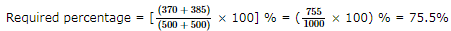SSC CPO Mock Test (Quant Apt) 5 - Question 45

In a school the periodical examinations are held every second month. In a session during April 2001-March 2002, a student of Class IX appeared for each of the perodical exams. The aggregate marks obtained by him in each periodical exam are represented in the line graph given below. Study the graph and answer the question based on it given below.
Marks obtained by a student in six periodical exams held in every two months during the years in the session 2001-02. Maximum total marks in each periodical exam = 500.Q. In which periodical exams there is a fall in percentage of marks as compared to the previous periodical exams ?

Detailed Solution for SSC CPO Mock Test (Quant Apt) 5 - Question 45

As is clear from the graph, the total marks obtained in periodical exams, go on increasing.
Since, the maximum marks for all the periodical exams are the same; it implies that the percentage of marks also goes on increasing.
Thus, in none of the periodical exams, there is a fall in percentage of marks compared to the previous exam.

SSC CPO Mock Test (Quant Apt) 5 - Question 46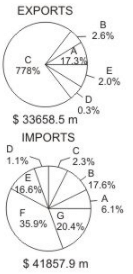Above circle graph shows the composition of Exports and Imports percent during 1998-99. Study the graph carefully and answer the questions.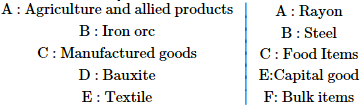Q. India's trade balance during the year 1998-99 was

Detailed Solution for SSC CPO Mock Test (Quant Apt) 5 - Question 46

As observable from the above pie-charts, imports are greater than exports.
∴∴ India's trade balance is unfavourbale

SSC CPO Mock Test (Quant Apt) 5 - Question 47Above circle graph shows the composition of Exports and Imports percent during 1998-99. Study the graph carefully and answer the questions.Q. Maximum export contribution has been due to

Detailed Solution for SSC CPO Mock Test (Quant Apt) 5 - Question 47

As observable from the graph, maximum export contribution is due to Manufactured goods

SSC CPO Mock Test (Quant Apt) 5 - Question 48Above circle graph shows the composition of Exports and Imports percent during 1998-99. Study the graph carefully and answer the questions.Q. The import of capital goods and other bulk items incurred nearly ___ of total expenditure.

Detailed Solution for SSC CPO Mock Test (Quant Apt) 5 - Question 48

Required % = (16.6 +35.9)% = 52.5%

SSC CPO Mock Test (Quant Apt) 5 - Question 49Above circle graph shows the composition of Exports and Imports percent during 1998-99. Study the graph carefully and answer the questions.Q. The imports exceeded exports by about ____ millions.

Detailed Solution for SSC CPO Mock Test (Quant Apt) 5 - Question 49

Imports exceeded by US \$ (41857.9-33650.5) million ≈ US \$ 8200 million

SSC CPO Mock Test (Quant Apt) 5 - Question 50Above circle graph shows the composition of Exports and Imports percent during 1998-99. Study the graph carefully and answer the questions.Q. The export of agriculture and allied products was to the tune of US\$.

Detailed Solution for SSC CPO Mock Test (Quant Apt) 5 - Question 50

Export of agricultural and allied products (in US\$)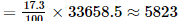## SSC CPO & Constable - Mock Tests & Previous Year Papers

1 docs|63 tests
 Use Code STAYHOME200 and get INR 200 additional OFF Use Coupon Code
Information about SSC CPO Mock Test (Quant Apt) 5 Page
In this test you can find the Exam questions for SSC CPO Mock Test (Quant Apt) 5 solved & explained in the simplest way possible. Besides giving Questions and answers for SSC CPO Mock Test (Quant Apt) 5, EduRev gives you an ample number of Online tests for practice

1 docs|63 tests